### Chemical and Process Engineering Resources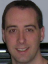## Basics of Industrial Heat Transfer

Nov 08 2010 01:30 PM | Chris Haslego in Heat Transfer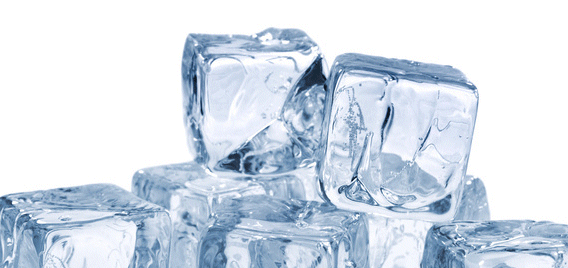Heat transfer is one of the most important industrial processes. Throughout any industrial facility, heat must be added, removed, or moved from one process stream to another. Understanding the basics of the heart of this operation is key to any engineers' mastery of the subject.

There are three basic types of heat transfer: conduction, convection, and radiation. The two most common forms encountered in the chemical processing industry are conduction and convection. This course will focus on these key types of heat transfer.

In theory, the heat given up by the hot fluid is never exactly equal to the heat gained by the cold fluid due to environmental heat losses. In practice, however, they are generally assumed to be equal to simplify the calculations involved. Any environmental losses are generally minimized with insulation of equipment and piping.

Any overall energy balance starts with the following equations: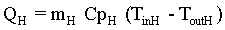Eq. (1)Eq. (2)

Where:

Q = heat transferred in thermal unit per time (Btu/h or kW)
M = mass flow rate
T = temperature
Cp = heat capacity or specific heat of fluid
Subscript "H" = hot fluid
Subscript "C" = cold fluid
When examining industrial systems, it is common practice to use a graphical form of these equations know as "T-Q diagrams" to enhance understanding and to make sure that the Second Law of Thermodynamics is not disobeyed. In other words, heat can only move from a higher to a lower temperature fluid. Here is how the generic diagram is constructed: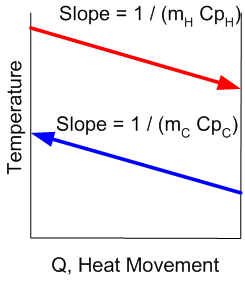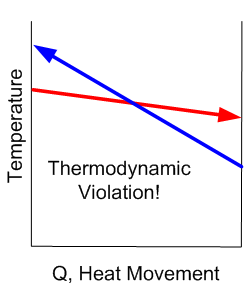Figure 1: Correct Example of a T-Q Diagram Figure 2: Incorrect Example of a T-Q Diagram

It's easy to see how viewing a particular heat transfer problem in this way is extremely valuable.
Now that's we've seen how heat moves from a hot fluid to a cold fluid, let's examine the third basic equation that is used to govern the equipment used for transferring heat.
The "Heat Exchanger Equation" takes the form:Eq. (3)

Where:
Q = heat transferred in thermal unit per time (Btu/h)
f = temperature correction factor
U = overall heat transfer coefficient (Btu/h ft2 Â°F)
A = heat transfer area (ft2)
LMTD = log mean temperature difference
These three (3) equations are the basis for virtually all heat exchanger design.

### Heat Transfer Articleschengineer

Heat Exchanger iOS application

https://itunes.apple...633422031?mt=8#!

I think it may be helpful to introduce an application (Heat Exchanger) which can be used in solving heat transfer equation and designing double pipe heat exchanger with following features:

1. Calculating each variable in energy balance equation
2. Designing double pipe heat exchanger with real size of pipe
3. Calculating overall heat transfer coefficient(U)
4. User can define any fluid with specific properties or select from most common fluids used in industrial application
5. Data can be edited in both SI and USCS unit standards.
6. User friendly UI designdebu

THANKS FOR THE ARTICLE. IF YOU SO KIND TO GIVE THE INFORMATION ABOUT DIRECT CONTACT JUICE HEATERS CALCULATION. THE HEATERS MAINLY USED IN SUGAR INDUSTRIES TO TEMPERATURE THE JUICE.monak

Thanks

### Forum Quick Links

•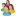Industrial Forum

•Student Forum

•Refining Forum

•Simulation Forum

•Relief Devices Forum

•Tank Venting Forum

• ### Article Navigation

•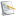Separation Technology

•Calculations and Tips

•Fluid Flow

•Heat Transfer

•Maintenance and Repair

•Utilities

•Safety and Pressure Relief

•Bulk Solids

•Process and Reactions

•Energy

•Other Topics

•For Students

•Physical Properties

•Archived Articles